# Important Objective questions on rotation for JEE Main/Advanced,CBSE,AP Physics

In this page we have Important Objective questions on rotation for JEE Main/Advanced,CBSE,AP Physics . Hope you like them and do not forget to like , social share and comment at the end of the page.
Question
Three bodies A(ring) ,B(Solid cylinder) ,C (solid sphere) rolls down the same inclined plane of angle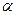and height  H  with out slipping.
The radii of the bodies are identical and equal to R.The mass of the bodies are
M1, M2, M3 respectively. The relation in the masses is given as below
M1 > M2 > M3
1 Statement I: The velocity of the center of mass of the bodies at the bottom of the incline plane is dependent on the mass of the bodies
Statement II: The total kinetic energy( Translation + Rotational) will be largest for the body A at the bottom of the inclined plane
a) Statement I is true only
b) Statement II is true only
c) Both the statements are true
d) Both of them are false
2. Which one will have largest center of mass velocity at the bottom of the incline plane
a)  A
b) B
c) C
d) Data insufficient
3 Which one will have least center of mass velocity at the bottom of the incline plane
a)  A
b) B
c) C
d) Data insufficient
4 At any time during the motion, the velocity of the point in contact of the body with the incline plane
a) 0
b) 2vcm
c) vcm
d) None of the above
5 At any time during the motion, the velocity of the uppermost point of the body
a) 0
b) 2vcm
c) vcm
d) None of the above
6 The angular velocity will be maximum at the bottom of the incline place for the body
a)  A
b) B
c) C
d) Data insufficient
Question
A nearly mass less rod (AB) of Length L is pivoted at one end A so that it can swing free as a pendulum. Three masses A(m) ,B(2m) and C( 3m) are attached to it at the distance L/4,L/3 and L/2 respectively from end A. The rod is held horizontal and then it is released
7 Find the Moment of the Inertia of system about the pivoted end
a)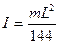b)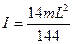c)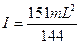d)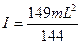8 Find the angular acceleration of the rod at the instant it is release
a)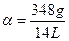b)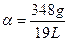c)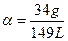d)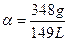Question
A projectile of mass m is fired from point O(origin) with an initial velocity u at angle θ above the horizontal
9 Find the X,Y,Z component of the angular momentum of the projectile as a function of time  about the origin
a) 0,0,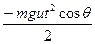b) 0,0,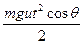c)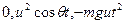d)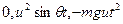10 Calculate the  torque of the weight acting on the projectile about the origin
a)  mgucos θ t k
b) - mgucos θ t k
c) - mgucos θ t j
d) none of these
11 Position vector of a body of mass 6 kg is given by as
r = i(3t2-6t) + j(-4t3)   m
Find the torque acting on it about the origin
a) k(-288t3+864 t2)
b) k(-288t3-864 t2)
c) 288 i + 864t2 j
d) None of these
Question
A rod of length L and mass M can freely rotate around a pivot at A. A bullet of mass m and velocity v hit the rod at a height h from A and becomes imbedded in it
12. Find the angular velocity of the Rod just after the collision
a)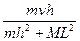b)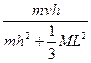c)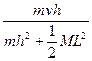d) None of these
13 Find the linear momentum of the system just after the collision if ω is the angular velocity just after the collision
a)(mh+ML/2) ω
b) (mh+ML) ω
c) (mh+ML/3) ω
d) None of these
Question
An upright hoop is projected on a pavement with a initial horizontal speed v0 but with out spin so that its slide. The resulting frictional force causes the hoop to spin to loose translational speed and to acquire an angular speed. Eventually the hoop rolls without slipping and let μ be the coefficient of friction
14 Find the time taken by the hoop to start rolling with out slipping
a) v0/2 μg
b) v0/2 g
c) v0/ μg
d) None of these
15 Find the velocity of the hoop at no slipping
a) v0/2
b)  3 v0/2
c) v0/4
d) None of these
1)  d
2) c
3) a
4) a
5) b
6) c
7) d
8) d
9) a
10) b
11) a
12) b
13) a
14) a
15) a

Solutions